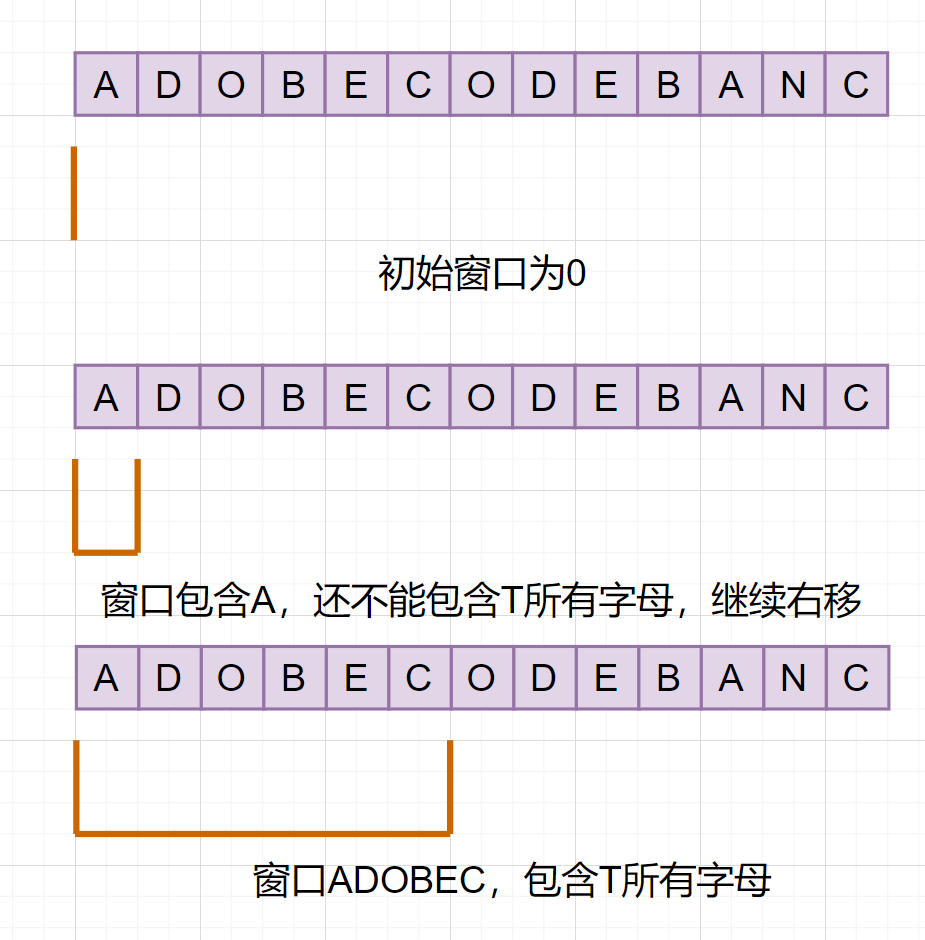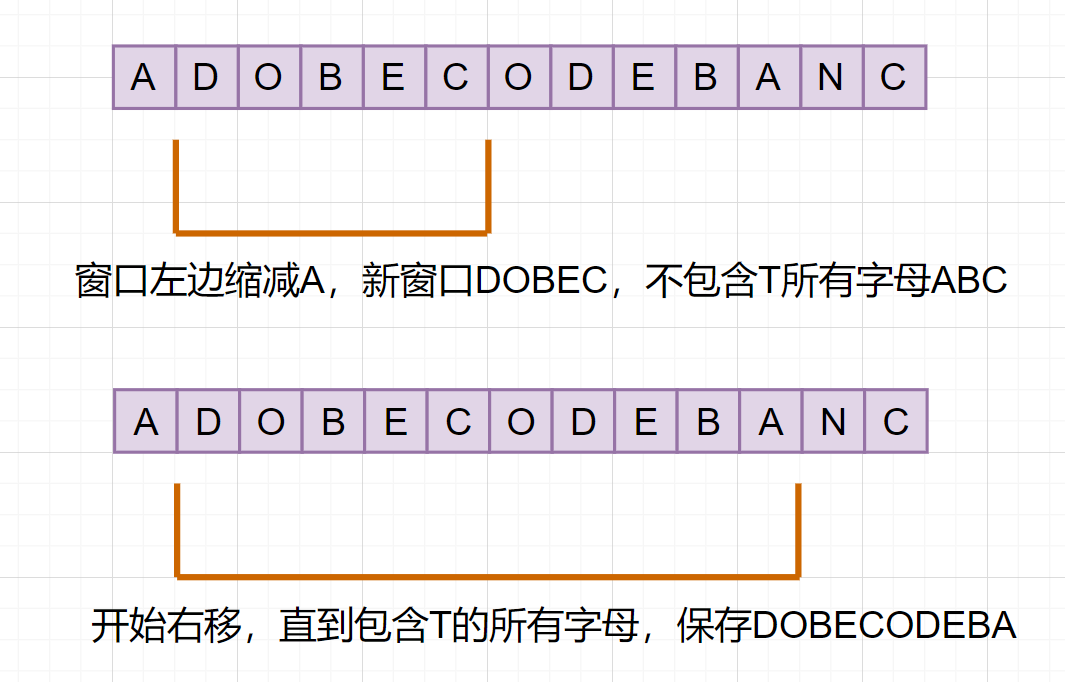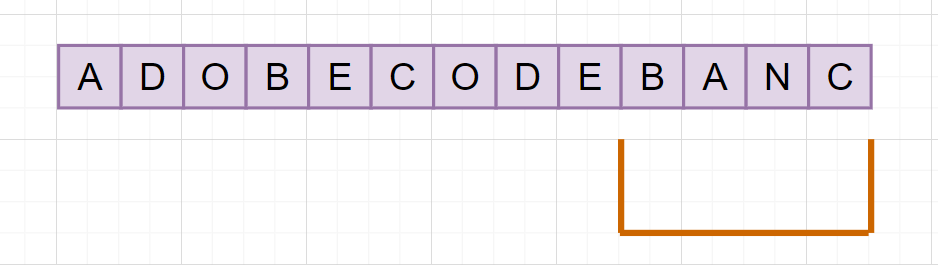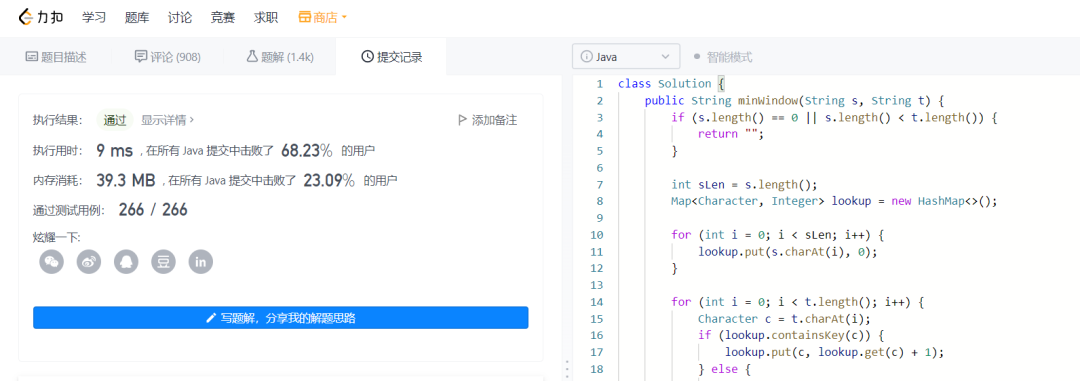# leetcode必备算法：聊聊滑动窗口

0收藏

## 前言

• 什么是滑动窗口？
• 一道算法题走进滑动窗口
• 滑动窗口可以用来解决哪些问题?
• 滑动窗口框架套路
• leetcode案例分析

## 什么是滑动窗口

TCP头部有个字段叫win，也即那个16位的窗口大小，它告诉对方本端的TCP接收缓冲区还能容纳多少字节的数据，这样对方就可以控制发送数据的速度，从而达到流量控制的目的。

TCP的滑动窗口在某一个时刻就是固定窗口大小的滑动窗口，随着网络流量等因素改变窗口大小也会随着改变。算法中的滑动窗口有点类似，就是维护一个窗口（队列/数组），不断滑动，然后更新答案。滑动窗口，指的是这样一类问题的求解方法，在数组上通过双指针同向移动而解决的一类问题。

## 一个例子走进滑动窗口算法

``````输入：arr [] = {100,200,300,400}  k = 2

``````   public int maxSum(int[] arry, int k) {
int size = arry.length;
int maxSum = 0;

for (int i = 0; i < size - k + 1; i++) {
int currentSum = 0;
for (int j = 0; j < k; j++) {
currentSum = currentSum + arry[i + j];
}

maxSum = Math.max(currentSum, maxSum);
}

return maxSum;
}``````

• 我们可以维护一个长度为2的窗口，初始化第一个窗口值的总和，并保存起来
• 然后窗口不断向右滑动，滑动过程中，与保存的最大值比较，并更新答案。
• 窗口直到滑到最右边才结束。

• 我们可以维护一个长度为3的窗口，初始化第一个窗口值的总和，并保存起来
• 然后窗口不断向右滑动，滑动过程中，与保存的最大值比较，并更新答案。
• 窗口直到滑到最右边才结束。

``````   public int maxSum1(int[] arry, int k) {
int size = arry.length;

if (size < k) {
return -1;
}

//初始化第一个窗口值的总和
int maxSum = 0;
for (int i = 0; i < k; i++) {
maxSum = maxSum + arry[i];
}

int sum = maxSum;
for (int i = k; i < size; i++) {
sum = sum + arry[i] - arry[i - k];
maxSum = Math.max(maxSum,sum);
}

return maxSum;
}``````

## 滑动窗口可以解决哪些问题

• 无重复字符的最长子串
• 最小覆盖子串
• 串联所有单词的子串
• 至多包含两个不同字符的最长子串
• 长度最小的子数组
• 滑动窗口最大值
• 字符串的排列
• 最小窗口子序列

## 滑动窗口框架套路

``````int left =0，right = 0;
while (right < s.size()){
//增大窗口
right++;

while (window needs shrink){
//缩小窗口
window.remove (s[left]);
left ++;
}
}``````

• 首先呢，就是获取原字符串的长度。
• 接着维护一个窗口（数组、哈希、队列）
• 窗口一步一步向右扩展
• 窗口在向右扩展滑动过程，需要判断左边是否需要缩减
• 最后比较更新答案

``````输入: s = "abcabcbb"

``````输入: s = "bbbbb"

``````int lengthOfLongestSubstring(String s){
//获取原字符串的长度
int len = s.length();
//维护一个哈希集合的窗口
Set<Character> windows = new HashSet<>();
int left=0,right =0;
int res =0;

while(right<len){
char c = s.charAt(right);
//窗口右移
right++;

//判断是否左边窗口需要缩减，如果已经包含，那就需要缩减
while(windows.contains(c)){
windows.remove(s.charAt(left));
left++;
}
//比较更新答案
res = Math.max(res,windows.size());
}
return res;
}``````

## leetcode案例分析

``````输入：s = "ADOBECODEBANC", t = "ABC"

``````输入：s = "a", t = "a"

``````- 首先呢，就是获取原字符串的长度。
- 接着维护一个窗口（数组、哈希、队列）
- 窗口一步一步向右扩展
- 窗口在向右扩展滑动过程，需要判断左边是否需要缩减
- 最后比较更新答案``````

### 获取原字符串的长度。

``int len = S.length();``

### 接着维护一个窗口（数组、哈希、队列）、右移、左边缩减• 1.当前窗口可能包含一个满足题目条件的，更小的子窗口字符串。（可以左边缩减）
• 2.窗口还没滑到的地方，可能包含一个满足条件的，更小的字符串。（可以窗口继续右移）

• 1.左边缩减，如果缩小的窗口仍然满足包含T所有字母，那当前窗口就可能是最小子串。存储下来（就类似于滑动窗口框架的更新答案哈），然后继续从左缩减窗口。
• 2.如果缩小窗口不能满足包含T的所有字母，这时候就可以停止窗口的左边缩减，转而向右扩大窗口啦。``````class Solution {
public String minWindow(String s, String t) {
if (s.length() == 0 || s.length() < t.length()) {
return "";
}

int sLen = s.length();
Map<Character, Integer> lookup = new HashMap<>();

for (int i = 0; i < sLen; i++) {
lookup.put(s.charAt(i), 0);
}

for (int i = 0; i < t.length(); i++) {
Character c = t.charAt(i);
if (lookup.containsKey(c)) {
lookup.put(c, lookup.get(c) + 1);
} else {
return "";
}
}

int left = 0;
int right = 0;
int minLen = Integer.MAX_VALUE;
int tCount = t.length();
String result = "";
while (right < sLen) {
char c = s.charAt(right);
if (lookup.get(c) > 0) tCount--;
lookup.put(c, lookup.get(c) - 1);
//窗口右移
right++;

//已经包含T的所有字母
while (tCount == 0) {
//比较更新答案
if (minLen > right - left) {
minLen = right - left;
result = s.substring(left, right);
}
char c2 = s.charAt(left);
if (lookup.get(c2) == 0) tCount++;
lookup.put(c2, lookup.get(c2) + 1);
//窗口从左边缩减
left++;
}
}
return result;
}
}``````

leetcode提交结果如下：-- end --帖子
视频
声望
粉丝
最近发布
相关问题
社区精华内容